Open in App
Not now

# Classifying Clothing Images in Python

• Last Updated : 13 Jan, 2022

Everywhere in social media image classification takes place from picking profile photos on Facebook, Instagram to categorizing clothes images in shopping apps like Myntra, Amazon, Flipkart, etc. Classification has become an integral part of any e-commerce platform. Classification is also used on identifying criminal faces in law and social networking.

In this article, we will learn how to classify images in Python. Classifying clothing images is an example of image classification in machine learning which means to classify the images into their respective category classes. For getting clothing images we will use the fashion_mnist dataset which comes with TensorFlow. This dataset contains clothing images of 10 different categories. It is a replacement for the beginner’s MNIST dataset which consists of handwritten digits. We will know more about it as we proceed.

## Stepwise Implementation

### Step 1: Importing the necessary libraries for the classification

• TensorFlow: To develop and train model using python
• NumPy: For array manipulation
• Matplotlib: For data visualization

## Python3

 `# importing the necessary libraries` `import` `tensorflow as tf` `import` `numpy as np` `import` `matplotlib.pyplot as plt`

Then we load the fashion_mnist dataset and we see the shapes of the training and testing data. It is evident that there are 60,000 training images to train the data and 10,000 testing images to test on the model. In total it contains 70,000 images in ten categories i.e ‘T-shirt/top’, ‘Trouser’, ‘Pullover’, ‘Dress’, ‘Coat’, ‘Sandal’, ‘Shirt’, ‘Sneaker’, ‘Bag’, ‘Ankle boot’.

## Python3

 `# storing the dataset path` `clothing_fashion_mnist ``=` `tf.keras.datasets.fashion_mnist`   `# loading the dataset from tensorflow` `(x_train, y_train),` `(x_test, y_test) ``=` `clothing_fashion_mnist.load_data()`   `# displaying the shapes of training and testing dataset` `print``(``'Shape of training cloth images: '``,` `      ``x_train.shape)`   `print``(``'Shape of training label: '``,` `      ``y_train.shape)`   `print``(``'Shape of test cloth images: '``,` `      ``x_test.shape)`   `print``(``'Shape of test labels: '``,` `      ``y_test.shape)`

Output: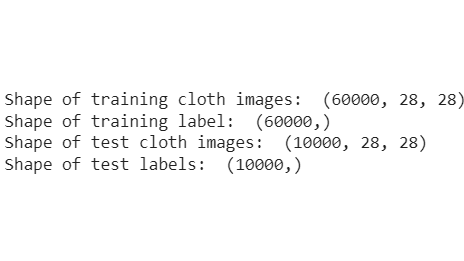The labels consist of an array of integers that ranges from 0 to 9. Since the class names are not added in the fashion_mnist dataset we then store the actual class names in a variable to use them later for data visualization. From the output, we can see that the pixel value fall in the range of 0 to 255.

## Python3

 `# storing the class names as it is` `# not provided in the dataset` `label_class_names ``=` `[``'T-shirt/top'``, ``'Trouser'``, ` `                     ``'Pullover'``, ``'Dress'``, ``'Coat'``,` `                     ``'Sandal'``, ``'Shirt'``, ``'Sneaker'``, ` `                     ``'Bag'``, ``'Ankle boot'``]`   `# display the first images` `plt.imshow(x_train[``0``])  ` `plt.colorbar()  ``# to display the colourbar` `plt.show()`

Output: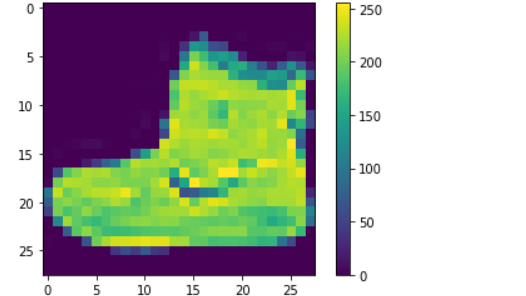### Step 3: Preprocessing the data

The below code normalizes the data as we can see that the pixel values fall in the range of 0 to 255. Hence we need to divide each by 255 to scale the value between 0 and 1.

## Python3

 `x_train ``=` `x_train ``/` `255.0`  `# normalizing the training data` `x_test ``=` `x_test ``/` `255.0`  `# normalizing the testing data`

### Step 4: Data Visualization

The below code displays the first 20 clothing images with their class labels making sure we are going in the right direction to build the model. Here we plotted x_train with colormap as binary and added the class names of each from the label_class_names array which we have stored previously.

## Python3

 `plt.figure(figsize``=``(``15``, ``5``))  ``# figure size` `i ``=` `0` `while` `i < ``20``:` `    ``plt.subplot(``2``, ``10``, i``+``1``)` `    `  `    ``# showing each image with colourmap as binary` `    ``plt.imshow(x_train[i], cmap``=``plt.cm.binary)` `    `  `    ``# giving class labels` `    ``plt.xlabel(label_class_names[y_train[i]])` `    ``i ``=` `i``+``1` `    `  `plt.show()  ``# plotting the final output figure`

Output: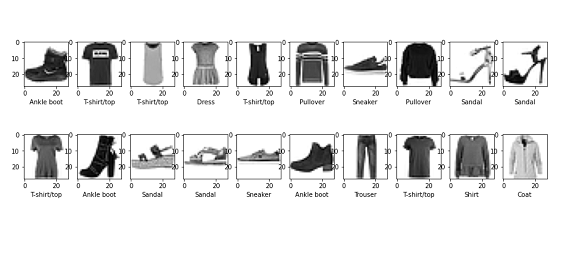### Step 5: Building the model

Here we build our model by creating layers of neural network. The tf.keras.layers.Flatten() converts the images from a two-dimensional array into a one-dimensional array and tf.keras.layers.Dense, have certain parameters that are learned during the training phase.

## Python3

 `# Building the model` `model ``=` `tf.keras.Sequential([` `    ``tf.keras.layers.Flatten(input_shape``=``(``28``, ``28``)),` `    ``tf.keras.layers.Dense(``128``, activation``=``'relu'``),` `    ``tf.keras.layers.Dense(``10``)` `])`

### Step 6: Compiling the model

Here we are compiling the model using adam optimizer, SparseCategoricalCrossentropy as the loss function, and metrics=[‘accuracy’].

## Python3

 `# compiling the model` `cloth_model.``compile``(optimizer``=``'adam'``,` `                    ``loss``=``tf.keras.losses.SparseCategoricalCrossentropy(` `                        ``from_logits``=``True``),` `                    ``metrics``=``[``'accuracy'``])`

### Step 7: Training the data on the model built

Now we will feed the x_train, y_train i.e the training data into our already compiled model. The model.fit() method helps in fitting the training data into our model.

## Python3

 `# Fitting the model to the training data` `cloth_model.fit(x_train, y_train, epochs``=``10``)`

Output: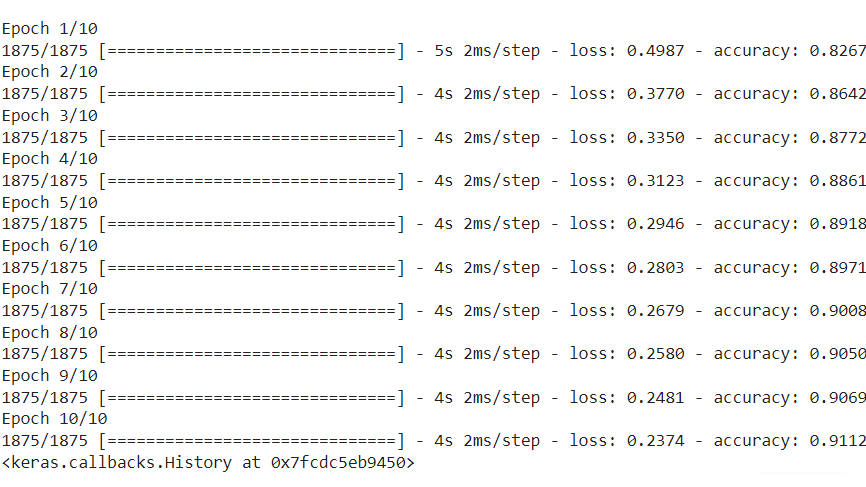### Step 8: Evaluate model loss and accuracy

Here we will see how good our model is by calculating the model loss and accuracy. From the output, we can see that the accuracy score on the testing data is less than that of training data. So it is an overfitted model.

## Python3

 `# calculating loss and accuracy score` `test_loss, test_acc ``=` `cloth_model.evaluate(x_test, ` `                                           ``y_test, ` `                                           ``verbose``=``2``)` `print``(``'\nTest loss:'``, test_loss)` `print``(``'\nTest accuracy:'``, test_acc)`

Output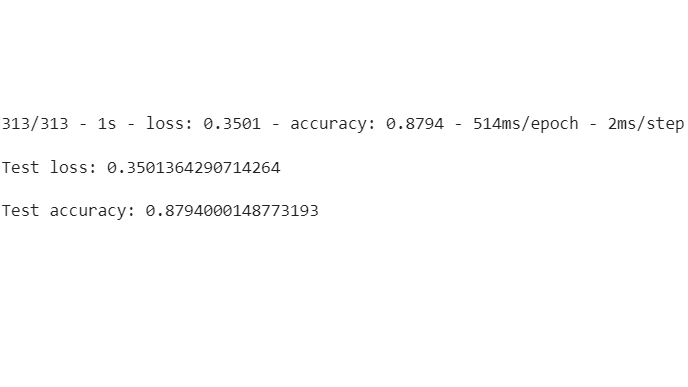### Step 9: Making predictions on trained model with test data

Now we can use the test dataset to make predictions on the model built. We tried to predict the first test image i.e x_test using predictions which resulted in test label 9 i.e Ankle boot. We have added the Softmax() function to convert the linear output logits to probability as it is much easier to calculate

## Python3

 `# using Softmax() function to convert` `# linear output logits to probability` `prediction_model ``=` `tf.keras.Sequential(` `    ``[cloth_model, tf.keras.layers.Softmax()])`   `# feeding the testing data to the probability ` `# prediction model` `prediction ``=` `prediction_model.predict(x_test)`   `# predicted class label` `print``(``'Predicted test label:'``, np.argmax(prediction[``0``]))`   `# predicted class label name` `print``(label_class_names[np.argmax(prediction[``0``])])`   `# actual class label` `print``(``'Actual test label:'``, y_test[``0``])`

Output: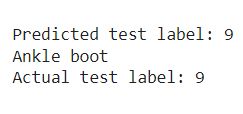### Step 10: Data Visualization of predicted vs actual test labels

At last, we will be visualizing our first 24 images predicted vs actual class labels to see how good our model is.

## Python3

 `# assigning the figure size` `plt.figure(figsize``=``(``15``, ``6``))` `i ``=` `0`   `# plotting total 24 images by iterating through it` `while` `i < ``24``:` `    ``image, actual_label ``=` `x_test[i], y_test[i]` `    ``predicted_label ``=` `np.argmax(prediction[i])` `    ``plt.subplot(``3``, ``8``, i``+``1``)` `    ``plt.tight_layout()` `    ``plt.xticks([])` `    ``plt.yticks([])` `    `  `    ``# display plot` `    ``plt.imshow(image)` `    `  `    ``# if else condition to distinguish right and ` `    ``# wrong` `    ``color, label ``=` `(``'green'``, ``'Correct Prediction'``) ` `    ``if` `predicted_label ``=``=` `actual_label ``else` `(` `        ``'red'``, ``'Incorrect Prediction'``)` `    `  `    ``# plotting labels and giving color to it` `    ``# according to its correctness` `    ``plt.title(label, color``=``color)` `    `  `    ``# labelling the images in x-axis to see` `    ``# the correct and incorrect results` `    ``plt.xlabel(``" {} ~ {} "``.``format``(` `        ``label_class_names[actual_label], ` `      ``label_class_names[predicted_label]))` `    `  `    ``# labelling the images orderwise in y-axis` `    ``plt.ylabel(i)` `    `  `    ``# incrementing counter variable` `    ``i ``+``=` `1`

Output:

As we can clearly see that the 12the, 17th, and 23rd predictions are wrongly classified but the rest is correct. Since no classification model can be 100% correct in reality this is a quite good model we built.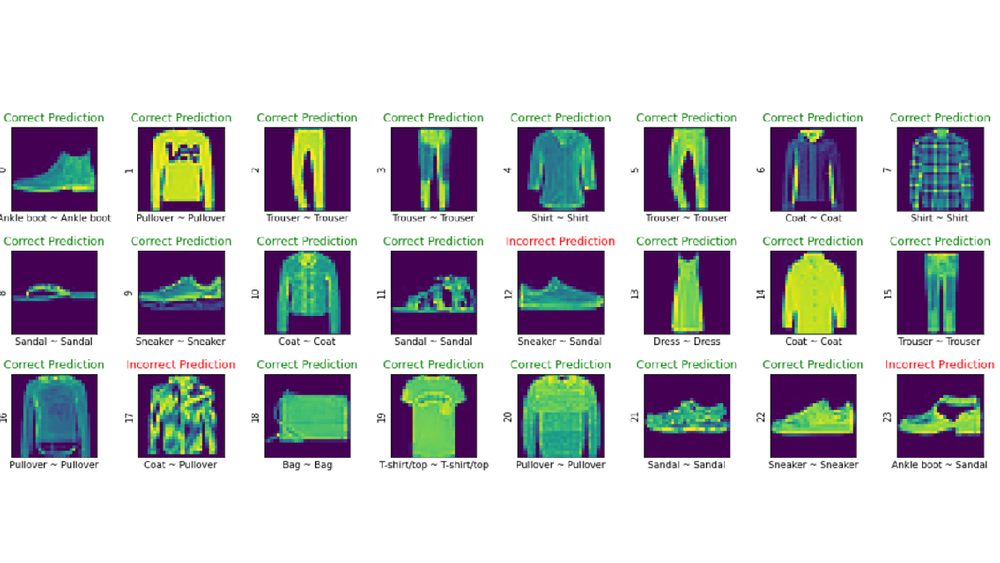My Personal Notes arrow_drop_up
Related Articles